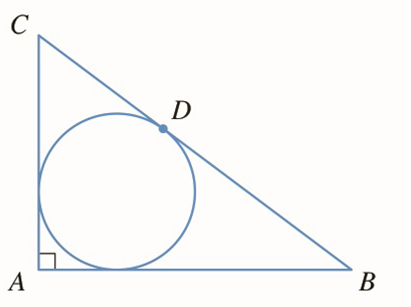Chapter 3.P, Problem 16P

Chapter
Section
Textbook Problem

# (a) Let ABC be a triangle with right angle A and hypotenuse a = | B C | .(see the figure.)If the inscribed circle touches the hypotenuse at D , show that | C D | = 1 2 ( | B C | + | A C | − | A B | ) (b) If θ = 1 2 ∠ C express the radius r of the inscribed circle in terms of a and θ .(c) If a is fixed and θ varies, find the maximum value of r .To determine

(a)

To show:|CD|=12BC+AC-AB

Explanation

1) Concept:

Use geometry theorems related to two tangents to a circle and point of tangency.

2) Given:

ABC be a triangle with right angle A and hypotenuse a=BC

3) Calculation:

A tangent to a circle is perpendicular to the radius drawn to the point of tangency.

So, quadrilateral AEOF is a square.

As the radius of circle is r, all sides of square are r.

So, AE=AF=r

So, |BE|=|AB|-r, |CF|=|AC|-r

Tangent segments to a circle from a point outside the circle are congruent.

So, |CF|=|CD|=|AC|-r

|BE|=|BD|=|AB|-r

12BC+AC-AB

= 12(BD+CD</

To determine

(b)

To express: The radius r  of inscribed circle in terms of a and θ

### Still sussing out bartleby?

Check out a sample textbook solution.

See a sample solution

#### The Solution to Your Study Problems

Bartleby provides explanations to thousands of textbook problems written by our experts, many with advanced degrees!

Get Started

#### In Exercises 1-6, simplify the expression. 5. (4x1)(3)(3x+1)(4)(4x1)2

Applied Calculus for the Managerial, Life, and Social Sciences: A Brief Approach

#### If f(x) = 2e3x, f(x) = a) 2e3x b) 2xe3x c) 6e3x d) 6xe3x

Study Guide for Stewart's Single Variable Calculus: Early Transcendentals, 8th

#### The area of the region bounded by , , and r = sec θ is:

Study Guide for Stewart's Multivariable Calculus, 8th